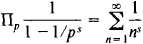# prime number

Also found in: Dictionary, Thesaurus.

## prime number:

see number theorynumber theory,
branch of mathematics concerned with the properties of the integers (the numbers 0, 1, −1, 2, −2, 3, −3, …). An important area in number theory is the analysis of prime numbers.
.

## Prime Number

(or prime), a positive integer that is greater than 1 and has no divisors other than itself and 1. For example, 2,3,5,7,11, and 13 are prime numbers.

The concept of prime number is basic to the study of the divisibility of the natural numbers, or positive integers. Thus, according to the fundamental theorem of arithmetic, any positive integer greater than 1 either is a prime or can be factored into a product of primes in one and only one way, apart from the order of the factors.

As long ago as the ancient Greek mathematicians it was known that there are infinitely many prime numbers; a proof can be found in the ninth book of Euclid’s Elements. Problems of the divisibility of the natural numbers and, consequently, problems associated with prime numbers are of importance in the study of groups. In particular, the structure of a group with a finite number of elements is closely related to the way in which the number of elements, or order of the group, decomposes into prime factors. Problems of the divisibility of integral algebraic numbers are considered in the theory of algebraic numbers.

Since the concept of prime number proved to be insufficient for the construction of a theory of divisibility, the concept of ideal was developed. In 1837, P. G. L. Dirichlet showed that the arithmetic progression a + bx, where x = 1,2,… and a and b are relatively prime integėrs, contains infinitely many prime numbers.

An extremely difficult problem in number theory is the distribution of the primes among the natural numbers. This problem involves the study of the asymptotic behavior of the function π(ξ), which is the number of primes not greater than the positive number x. The first results in this direction were obtained by P. L. Chebyshev, who proved in 1850 that there exist two constants a and A such thatfor all x2. In other words, π(x) increases as x/ln x. In 1896, J. Hadamard and C. J. de La Vallée Poussin made the next important step when they independently proved what is called the prime number theorem, which is a refinement of Chebyshev’s theorem. The prime number theorem states that the limit of the ratio of π(x) and x/ln x, as x becomes infinite, is 1.

Mathematicians have since devoted considerable effort toward refining the prime number theorem. The distribution of the prime numbers have been studied by means of both elementary methods and the methods of mathematical analysis. A particularly fruitful method is based on the identitywhere the product is taken over all the primes ρ = 2,3, — This identity was introduced by L. Euler. It holds for all complex s whose real part is greater than 1. The identity is the basis for the study of the distribution of the prime numbers through the use of special functions called zeta functions ζ (s). For Re s > 1, the function is defined as the seriesThis function was used for real values of s by Chebyshev to study the distribution of the prime numbers. G. F. B. Riemann pointed out the importance of studying ζ(s) for complex values of 5. Riemann put forth the conjecture that all the roots of the equation ζ(s) = 0 in the right half plane have their real parts equal to ½. As of 1975, this conjecture had not been proved. Its proof would be an important step toward the solution of the problem of the distribution of the prime numbers. The distribution of the prime numbers is closely related to the Goldbach problem, the still unsolved problem of twin primes, and other problems in analytic number theory. The problem of twin primes is whether the number of prime numbers differing by 2, for example, 11 and 13, is finite or infinite. Tables of the prime numbers among the first 11,000,000 natural numbers show the existence of very large twin primes, for example, 10,006,427 and 10,006,429. This fact, however, does not prove that there are infinitely many such pairs. A number of primes outside the range of existing tables admit of a simple arithmetic expression. For example, it was shown in 1965 that 211,213 - 1 is a prime number; this number contains 3,376 digits.

### REFERENCES

Vinogradov, I. M. Osnovy teorii chisel, 8th ed. Moscow, 1972.
Hasse, H. Lektsii po teorii chisel. Moscow, 1953. (Translated from German.)
Ingham, A. E. Raspredelenie prostykh chisel. Moscow-Leningrad, 1936. (Translated from English.)
Prachar, K. Raspredelenie prostykh chisel. Moscow, 1967. (Translated from German.)
Trost, E. Prostye chisla. Moscow, 1959. (Translated from German.)

## prime number

[′prīm ′nəm·bər]
(mathematics)
A positive integer having no divisors except itself and the integer 1.

## prime number

an integer that cannot be factorized into other integers but is only divisible by itself or 1, such as 2, 3, 5, 7, and 11

## prime number

An integer greater than 1 that is not evenly divisible by any number other than itself, and, of course, 1. Prime numbers are considered a fundamental building block of numbers, especially number theory, a branch of mathematics.

The first 10 prime numbers are 2, 3, 5, 7, 11, 13, 17, 19, 23 and 29. Except for 2, all primes are odd numbers. As the numbers get higher, prime numbers tend to be found in pairs that are close together; for example, 29,669 and 29,671 are primes. The largest prime number thus far, which contains more than 65,000 digits, was discovered in 1986.
Site: Follow: Share:
Open / Close